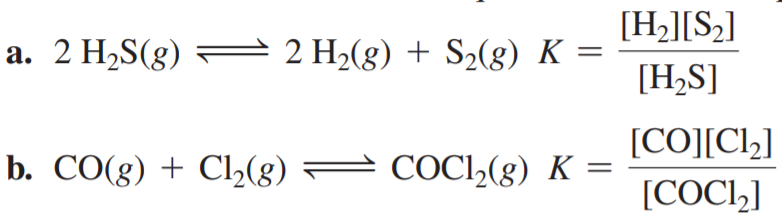×
Get Full Access to Chemistry: A Molecular Approach - 3 Edition - Chapter 14 - Problem 22e
Get Full Access to Chemistry: A Molecular Approach - 3 Edition - Chapter 14 - Problem 22e

×

# Find and fix each mistake in the equilibrium constantISBN: 9780321809247 1

## Solution for problem 22E Chapter 14

Chemistry: A Molecular Approach | 3rd Edition

• Textbook Solutions
• 2901 Step-by-step solutions solved by professors and subject experts
• Get 24/7 help from StudySoup virtual teaching assistantsChemistry: A Molecular Approach | 3rd Edition

4 5 1 339 Reviews
21
5
Problem 22E

Find and fix each mistake in the equilibrium constant expressions.Step-by-Step Solution:

Solution 22EStep 1In general. Chemical equilibrium can be represented asWhere A and B are the reactants and C and D are the products and a. b. c. and d are thestoichiometric coefficients respectively. According to the Law of mass action, rate of forwardreaction is equal to the rate of backward reaction, therefore equilibrium constant (K) can begiven as the ratio of concentration of products raised to the power of its stoichiometriccoefficient to the concentration of reactant raised to the power of its coefficients that is:

Step 2 of 3

Step 3 of 3

##### ISBN: 9780321809247

Unlock Textbook Solution